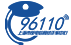• 上证- - -(涨:-平:- 跌:-)深证- - -(涨:-平:- 跌:-)

-
- -
• 今开:-
• 最高:-
• 涨停:-
• 换手:-
• 成交量:-
• 振幅:-
• 外盘:-
• 昨收:-
• 最低:-
• 跌停:-
• 量比:-
• 成交额:-
• 均价:-
• 内盘:-• 主要指标
• 均线
• EXPMA
• BOLL
• SAR
• BBI
• RSI
• KDJ
• MACD
• W&R
• DMI
• BIAS
• OBV
• CCI
• ROC
• CR
• BOLL
• RSI
• KDJ
• MACD
• W&R
• DMI
• BIAS
• OBV
• CCI
• ROC
• CR
• BOLL
 封基排行 - (- -) - (- -) - (- -) - (- -) - (- -) - (- -)

### 石油基金吧